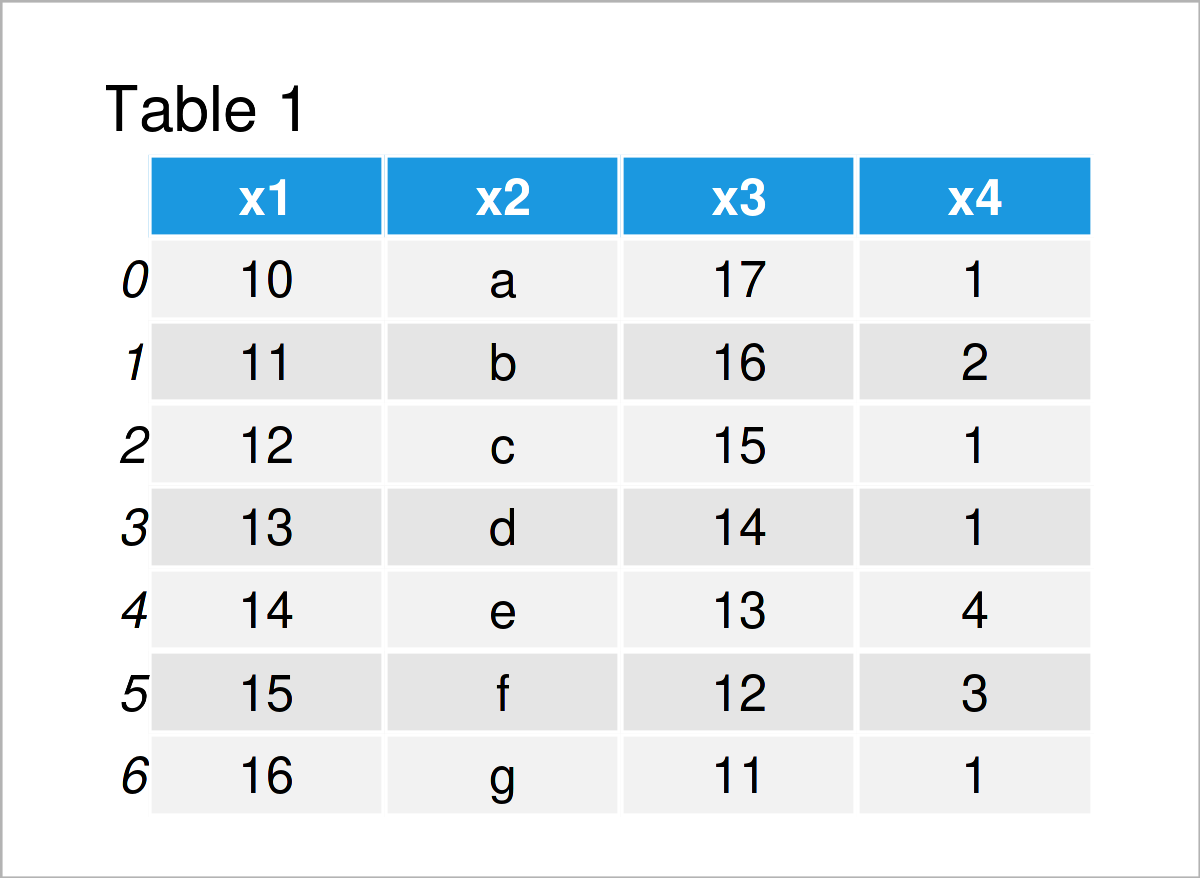# Create Subset of Rows of pandas DataFrame in Python (2 Examples)

In this Python programming tutorial you’ll learn how to extract a subset of pandas DataFrame rows.

The post consists of the following content:

Let’s dive right into the examples…

## Example Data & Libraries

We first have to import the pandas library:

`import pandas as pd                         # Import pandas`

As a next step, let’s also construct some example pandas DataFrame:

```data = pd.DataFrame({'x1':range(10, 17),    # Create pandas DataFrame
'x2':['a', 'b', 'c', 'd', 'e', 'f', 'g'],
'x3':range(17, 10, - 1),
'x4':[1, 2, 1, 1, 4, 3, 1]})
print(data)                                 # Print pandas DataFrame```Have a look at the previous table. It shows that our example data consists of seven rows and four columns.

## Example 1: Create Subset of pandas DataFrame Based on Logical Condition

This example demonstrates how to get a subset of rows of a pandas DataFrame using a logical condition.

Consider the Python syntax below:

```data_sub1 = data.loc[data['x4'] >= 2]       # Get rows in range
print(data_sub1)                            # Print DataFrame subset```By executing the previous Python programming code, we have created Table 2, i.e. a new pandas DataFrame containing only those rows of our input data set where the column x4 has a value larger than or equal to 2.

## Example 2: Create Random Subset of pandas DataFrame

This example demonstrates how to generate a random subsample of a pandas DataFrame in Python programming.

We first have to load the NumPy library to Python, if we want to apply the corresponding functions:

`import numpy                                # Import numpy`

Next, we can set a random seed to make our example reproducible:

`numpy.random.seed(735658)                   # Set random seed for reproducibility`

Finally, we can apply the sample function to our pandas DataFrame to generate a randomly selected subset of rows:

```data_sub2 = data.sample(frac = 0.5)         # Draw random DataFrame subset
print(data_sub2)                            # Print DataFrame subset```As shown in Table 3, we have created another pandas DataFrame containing only 50 percent of the rows of our input data set with the previous Python programming code.

## Video & Further Resources

Have a look at the following video on my YouTube channel. In the video, I explain the Python code of this article in the Python programming language:

Please accept YouTube cookies to play this video. By accepting you will be accessing content from YouTube, a service provided by an external third party.If you accept this notice, your choice will be saved and the page will refresh.

Furthermore, you might want to have a look at the related Python articles on this website. A selection of posts about topics such as counting, descriptive statistics, and extracting data is shown here:

To summarize: In this tutorial you have learned how to select a subset of pandas DataFrame rows in the Python programming language. If you have additional comments or questions, tell me about it in the comments. Besides that, don’t forget to subscribe to my email newsletter to receive updates on new tutorials.

Subscribe to the Statistics Globe Newsletter